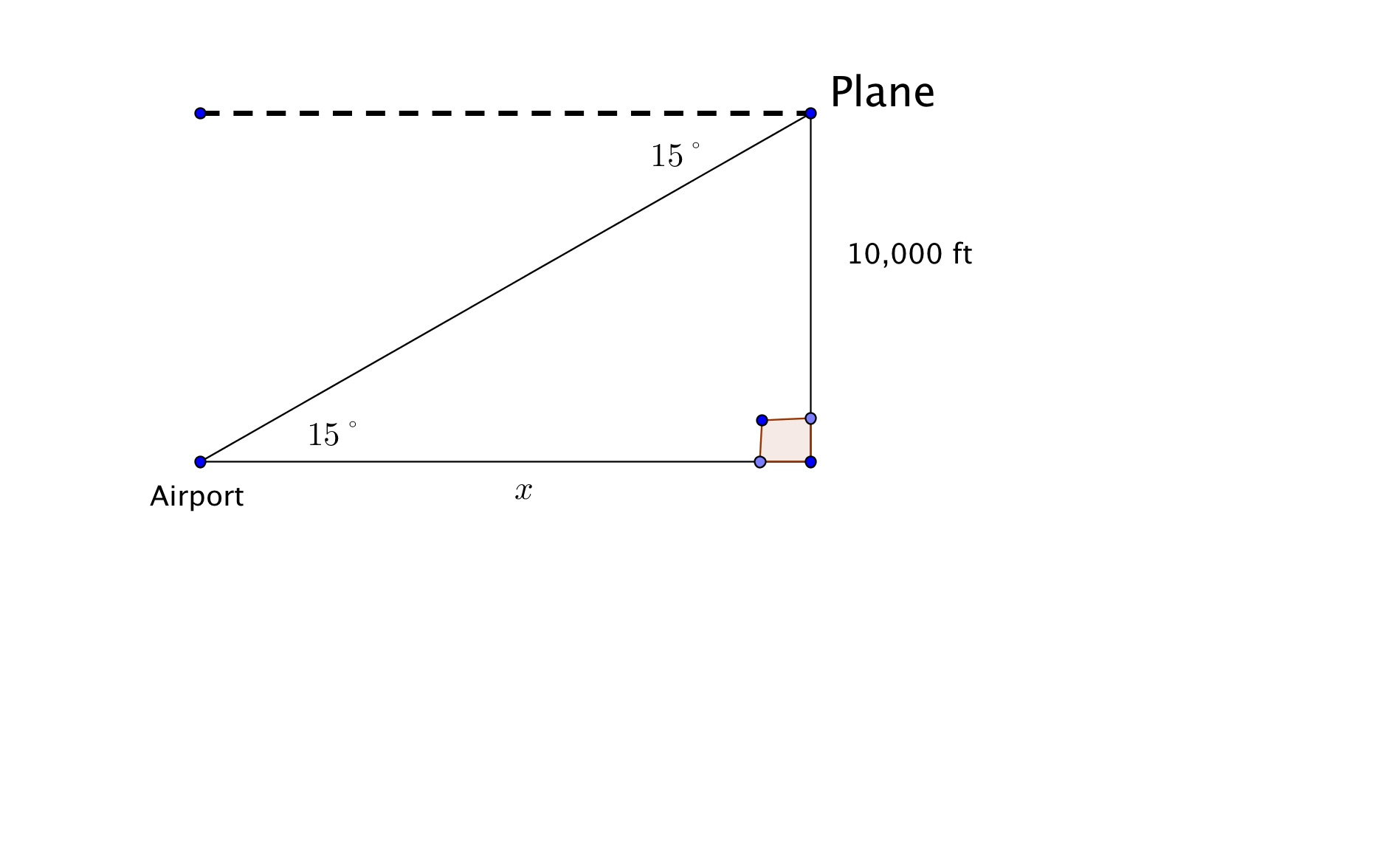# GEOM 2| Lesson 2| Practice 3 Solutions

1.$$tan 15˚ =\Large \frac{10,000}{x}$$

$$x = \Large\frac{10,000}{tan 15˚}$$  so $$x = 37,320.5$$ ft.

$$tan 12˚ = \Large\frac{12,000}{x}$$

$$x = \Large\frac{ 12,000}{tan 12˚}$$ or $$x = 56,455.6$$ ft

Using the pythagorean theorem, find $$y$$:  $$y = 57,716.8$$ ft

3.$$tan 35˚ = \Large\frac{x}{25}$$

$$x = 25 tan 35˚$$ ; $$x = 17.5$$ ft

But 5.5 needs to be added to it as the angle of elevation was taken from eye height.

The tree is $$23$$ft.